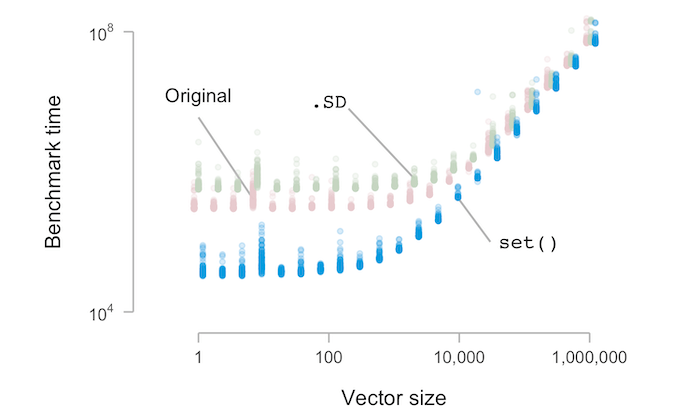Want to share your content on R-bloggers? click here if you have a blog, or here if you don't.

In a post last week I offered a couple of simple techniques for randomly shuffle a data.table column in place and benchmarked them as well. A comment on the original question, though, argued these timings aren’t useful since the benchmarked data set only contains five rows (the size of the table in the original post).

That seemed plausible, so I’ve carried the test further. Often we’re interested in vectors with hundreds, thousands, or millions of elements, not a handful. Do the timings change as the vector size grows?

To find out, I simply extended my computation from last time using microbenchmark and plotted the results below. I’m surprised to see just how much `set()` continues to outperform the other options even to fairly large vector sizes.Benchmark Code
```library(data.table)
library(microbenchmark)

scramble_orig <- function(input_dt, colname) {
new_col <- sample(input_dt[[colname]])
input_dt[, c(colname) := new_col]
}

scramble_set <- function(input_dt, colname) {
set(input_dt, j = colname, value = sample(input_dt[[colname]]))
}

scramble_sd <- function(input_dt, colname) {
input_dt[, c(colname) := .SD[sample(.I, .N)], .SDcols = colname]
}

times <- rbindlist(
lapply(
setNames(nm = 2 ** seq(0, 20)),
function(n) {
message("n = ", n)
setDT(microbenchmark(
orig = scramble_orig(input_dt, "x"),
set  = scramble_set(input_dt, "x"),
sd   = scramble_sd(input_dt, "x"),
setup = {
input_dt <- data.table(x = seq_len(n))
set.seed(1)
},
check = "identical"
))
}
),
idcol = "vector_size"
)
```

Reading the chart from left to right, small vectors to large ones, the first regime is one where `set()` dominates the other methods, having a much shorter runtime. This is followed by a transition to a regime where the time required for `sample()` to shuffle large vectors dominates the run time. (Notice both axes are on the logarithmic scale, so the time is exponentially increasing.)

Does this matter? The differences here are so small that we can’t even use profvis to benchmark a single run. But, what if we were calling this functionality repeatedly in a loop? The differences add up.

This is a good example of where it’s nice to know the options available to us in the languages and packages being used: The data.table authors built `set()` for these kinds of reasons, as a way to programmatically assign to data.tables in place within loops.

In a one-off analysis, maybe it’s not worth the trouble to care too much about speed, and it’s likely not a good use of time to benchmark everything. But when writing packaged code, for example, we give up the ability to know how and where our code will be used. It pays to be aware of things like the difference between using `.SD` and `set()` and which is the better option. It makes our code more easily used in places we’d never thought about and can’t think about at the time.